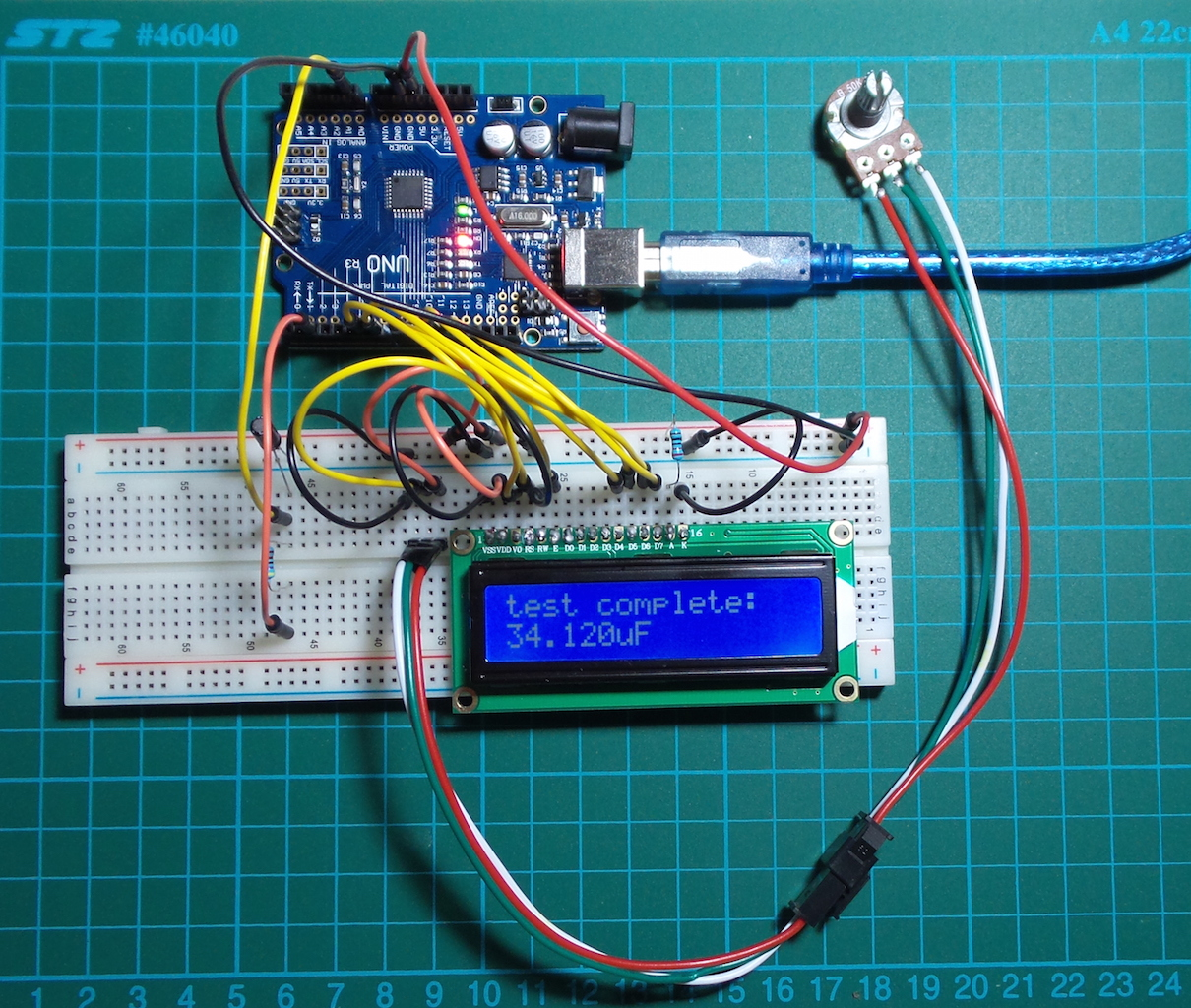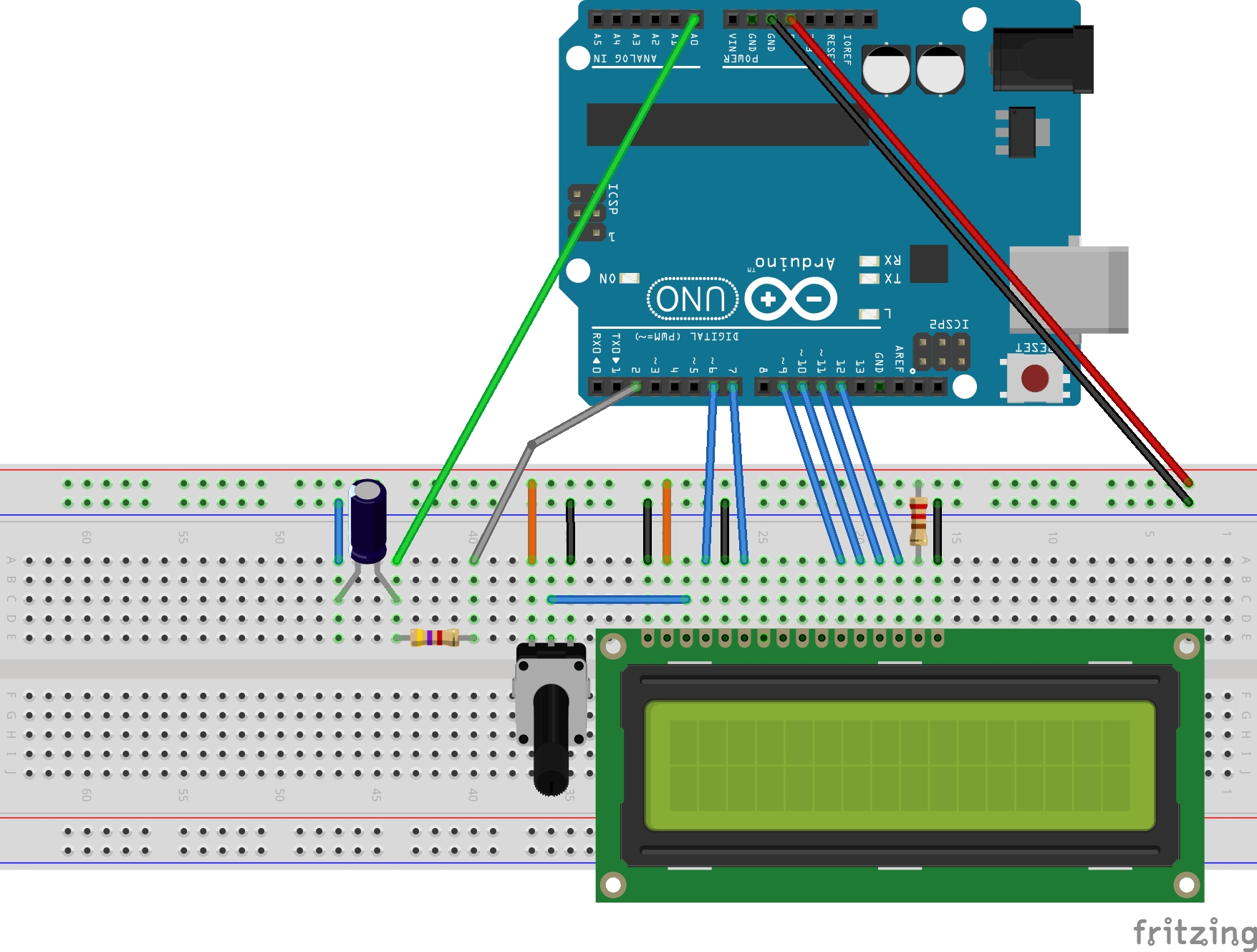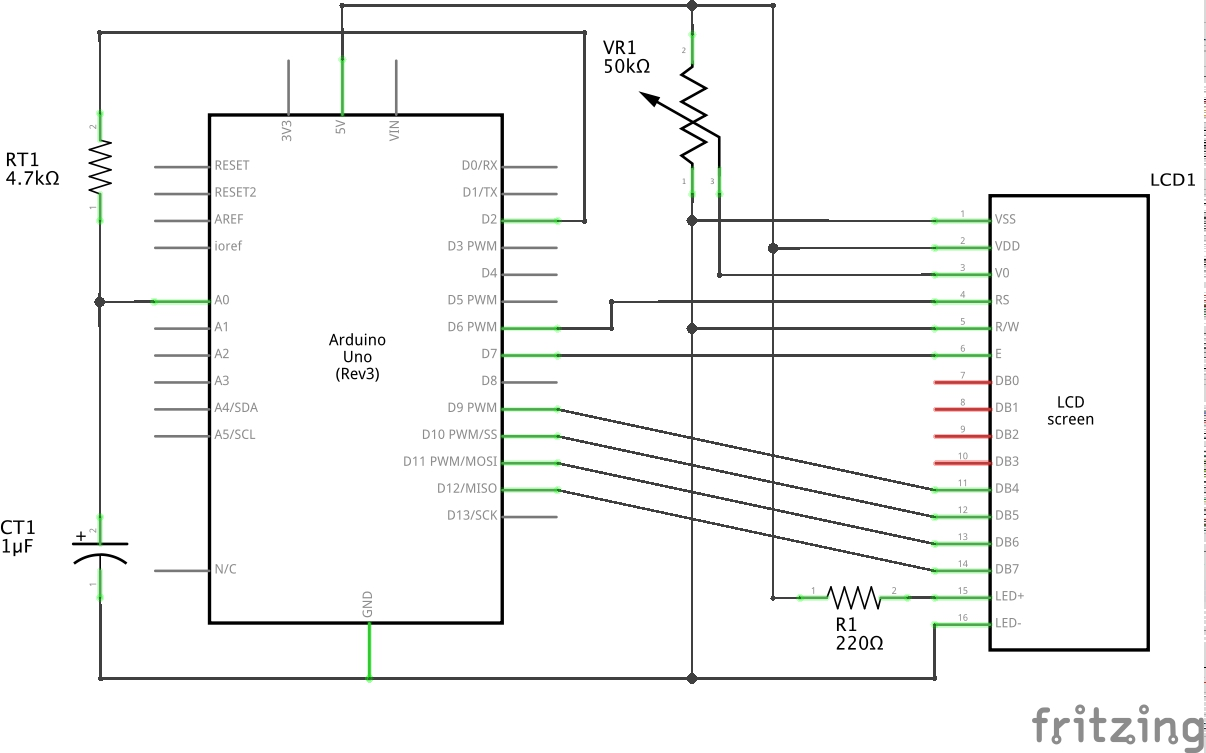#057 Capacitance Tester

Use an Arduino to test capacitors and output result to 16x2 LCD module.This circuit tests capacitance by measuring the RC time constant. It exploits two facts:

• the time constant is approximately the time required to charge the capacitor, through the resistor, to ≈ 63.2% of the difference between the initial value and final voltage
• the time constant = R x C

For this to work, we need three things:

• accurate measurement of the starting and final voltages
• accurate measurement of the resistance in the RC circuit
• accurate measurement of the time it takes to charge to 63.2% final voltage

Starting Voltage

We force this to 0V, by discharging the capacitor under test until V=0. For simplicity, the circuit discharges through the same pin as used for charging

Final Voltage

We are charging the capacitor with a digital output pin of the Arduino. Nominally, this should mean the final voltage is 5V, however not all Arduino boards will hit this accurately. We measure the actual 5V reference voltage and set the `vref_mv` constant in the sketch accordingly (4.93V in my case)

Actual Resistance (RT1)

The resistor RT1 should be measured with an ohmeter, and set the `rt1` constant in the sketch accordingly. Choosing a value for RT1 is a compromise between:

• making it small enough so that charge/discharge cycles are not unbearably long
• making it large enough so that an accurate measurement of the time constant is possible

In practice, I’ve settled on two values:

• 22k as a decent choice for capacitors between 10nF and 330uF
• 4.7k for capacitors over 330uF

Measuring the time constant

During the charge cycle, the voltage across the capacitor is measured with analog pin A0. When this hits the pre-calculated 63.2% level, the time elapsed is our measurement of the time constant. From this, the capacitance can then be calculated.

The standard microsecond counter `micros()` is used to measure timing. As stated in the micros() documentation this provides a precision of 4-8 microseconds, depending on the board:

• On 16 MHz Arduino boards (e.g. Duemilanove and Nano), this function has a resolution of four microseconds
• On 8 MHz Arduino boards (e.g. the LilyPad), this function has a resolution of eight microseconds.

This seems to work reasonably well for capacitors over 100nF (10nF at a stretch), but I haven’t had any luck at the pF scale.

Results

The sketch works quite well for testing a series of capacitors. With everything running, just unplug one capacitor and replace it with another. It will fully discharge the capacitor, measure the time constant, and print the capacitance to the LCD.

Type Nominal Measured
ceramic 10nF 16.667nF
ceramic 100nF 94.444nF
electrolytic 1uF 1.064uF
electrolytic 10uF 11.069uF
electrolytic 22uF 25.503uF
electrolytic 33uF 34.120uF
electrolytic 47uF 49.312uF
electrolytic 100uF 105.794uF
electrolytic 220uF 234.546uF
electrolytic 330uF 367.811uF

These measurements were taken using a 22k resistor for RT1. Note:

• the 10nF measurement is sketchy. The charge cycle is getting a bit short at that scale for much accuracy
• above 330uF, charge/discharge times are getting long. They work better with 4.7k resistor for RT1
• care is required to test with the correct polarity for electrolytics

LCD Output

Output is sent to an 16x2 LCD display. The LiquidCrystal library works with all LCD displays that are compatible with the Hitachi HD44780 driver. There are many of them out there, and you can usually tell them by the 16-pin interface.

A variable resistor is used to trim contrast, and the LCD backlight is powered from the 5V rail.

Construction